# High Efficiency DDR Termination Power Supplies Source and Sink More than 10 Amps

Introduction

The emerging standard for system memory in computing and communication systems is Double Data Rate (DDR) memory. Typical DDR memory needs at least two main power supplies: VDD and VTT, where VDD powers the I/O, and VTT powers the I/O termination. To ensure good signal quality and fast data rate, the termination power supply VTT must always track the VDD supply with VTT = VDD/2. Since the termination resistors can carry current in either direction, the VTT power supply must be able to both source and sink current while tracking the VDD supply. A typical DDR termination power supply for a PC application requires 2A to 10A of current. In servers, high power workstations or broadband networking equipment applications, the DDR termination supply current may well exceed 10A. At these current levels, a linear regulator is not a viable solution because of its substantial power losses; a synchronous buck converter topology is more appropriate.

With these DDR requirements in mind, Analog Devices has developed a family of new termination/tracking controllers, including the LTC3717, LTC3718 and LTC3831.

Two Basic Design Schemes

Figure 1 shows two basic design schemes for the termination power supplies. In scheme 1 VTT is generated from the VDD rail directly. Although popular in low power (<10A) DDR applications, this scheme has several disadvantages for high power applications:

• The power rating of the VDD supply must be able to provide enough current to power both VTT circuit and the I/O current requirement of the DDR memory. This results in higher system thermal stress, a higher solution cost (especially for the VDD supply) and lower system power conversion efficiency.
• Because the input ripple current of the VTT supply is pulsating (see Figure 1), the input caps on the VDD rail must have high RMS current capability and low impedance to minimize the VDD rail switching noise. Such caps add significant size and cost to the solution.Figure 1. Two basic design schemes for DDR termination power supplies.

A better solution for high power DDR supply applications, is Scheme 2, where VTT is generated from higher input voltage sources. Power losses are lower overall because the VDD supply output does not need to support VTT. The result is a smaller, cheaper and cooler power supply design. There is also no need to use additional caps on the VDD rail. The input source (VDC) for the VTT circuit must be able to sink current when the VTT output sinks current. It is desirable that this input voltage source of the VTT circuit also serves as the input source of the VDD supply. Consequently, any negative input current of the VTT circuit can be absorbed by the positive input current of the VDD supply.

Design Examples

The newly introduced LTC termination/tracking controller family: LTC3717, LTC3718 and LTC3831 can be applied to a variety of termination supply applications. In high current DDR supply applications, two of the versatile LTC1629 PolyPhase controllers can be used to generate both VDD and VTT.

High Efficiency 12A VTT Supply with Low Input Voltage (1.5V–5V) and Fast Transient Response

If the VTT is generated from VDD (scheme 1), which is currently 3.3V or 2.5V and trending toward 1.8V or 1.5V; or from a separate 3.3V system bus (Scheme 2), the input supply voltage (<5V) may not fully turn on the logic level MOSFETs, which require a 5V bias for the gate drive. If a 5V supply is unavailable, the LTC3718 has an integrated boost switcher to provide one. Figure 2(a) shows a complete schematic for a power supply that uses the LTC3718.Figure 2a. High efficiency 12A LTC3718 VTT power supply with 1.5V–5V input.

The LTC3718 is a No RSENSETM current mode synchronous buck controller that senses the operating current via the RDS(ON) of the bottom FET, eliminating the sense resistor and associated power loss. This improves efficiency and load transient response. The integrated 1.2MHz boost switcher generates the 5V output to efficiently drive the POWER MOSFETs. The input to the integrated boost switcher can be as low as 1.5V, making it possible to operate this circuit with any input between 1.5V and 5V. The VDD supply is used as a reference and is applied to the positive input terminal of the feedback error amplifier through an internal equal R value resistive divider. With this internal divider a regulation accuracy of 0.65% is possible without any additional external precision resistors. With only two small PowerPak SO-8 MOSFETs, and a 300kHz switching frequency, efficiency is better than 85% for a 2.5V input and 1.25V,12A output, as shown in Figure 2b.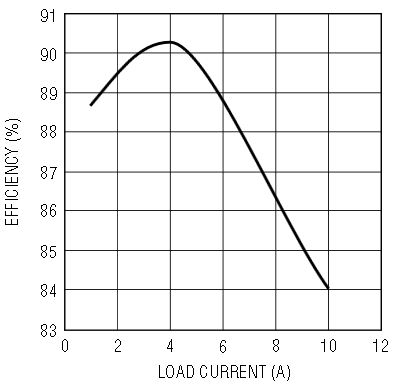Figure 2b. Efficiency vs load current for the circuit shown in Figure 2a, VIN = 2.5V, VTT = 1.25V.

High Efficiency 10A VTT Supply with Wide Input Voltage Range (5V–24V) and Fast Transient Response

If the input voltage range is between 5V and 24V, the LTC3717 provides the best compromise between performance and cost. Figure 3a shows a 10A design for a 2.5V VDD, 1.25V VTT, application using the LTC3717. If a 5V bias is already available for the MOSFET driver, this design can also be used for applications with an input voltage less than 5V.Figure 3a. High efficiency 10A LTC3717 VTT supply from 5V–24V input.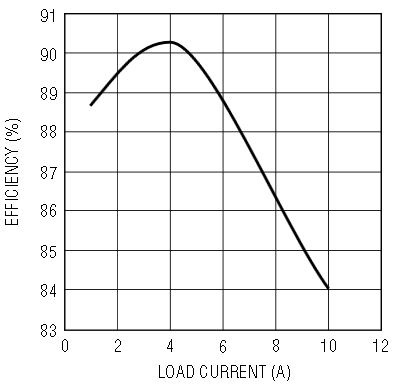Figure 3b. Efficiency vs load current for the circuit shown in Figure 3a, VIN = 12V, VTT = 1.25V.

The LTC3717 is similar to the LTC3718, except that it does not include a boost switcher. When more than 5V is applied to the VCC pin, the on-chip LDO generates enough current to drive logic-level MOSFETs. This design uses only two SO-8 PowerPak MOSFETs to deliver ±10A of output current. To provide higher output currents, use a higher current rated inductor and parallel more MOSFETs. At 250 KHz switching frequency, this circuit is 84% efficient at the 1.25V/10A output.

A unique feature of the LTC3717 is that the main controller implements the constant on-time architecture with the on-time programmed by the input voltage and RON. This scheme makes for a fairly constant, yet programmable, switching frequency while maintaining extremely fast load transient response, as seen in Figure 3c. With only two SP caps (270µF/2V), the VTT variation is about ±60mV with a 10A load step. The 60mV variation is mostly from the voltage dropped across the ESR of the output capacitors by the load step current. Figure 3d shows the voltage of VTT tracking a change in the voltage of VDD.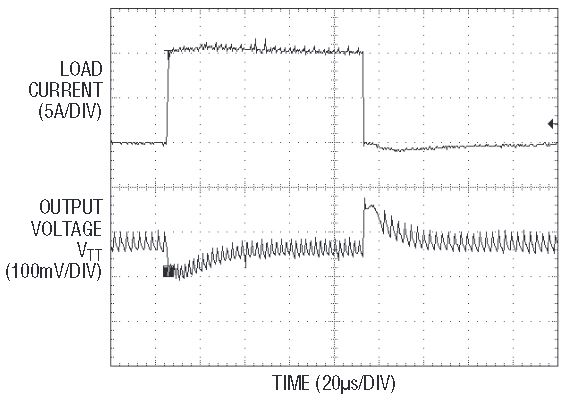Figure 3c. Load transient response for a 10A load applied to the circuit shown in Figure 3a VIN = 15V, VTT = 1.25V.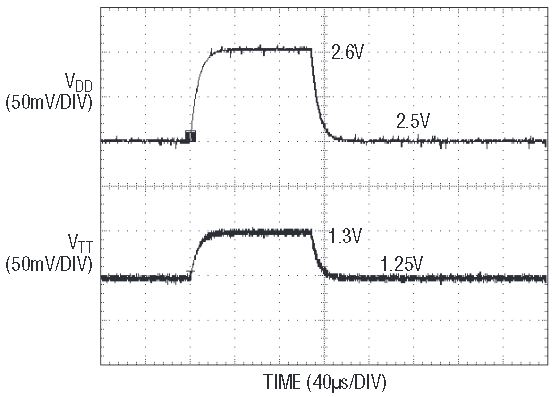Figure 3d. VTT responding to a change in VDD for the circuit shown in Figure 3a.

The LTC3717 controller also has a minimum on-time that is less than 100ns, enabling high step-down ratio applications at very high switching frequencies. For example, if the input voltage is 20V and the output voltage is 0.75V, a controller with a minimum on-time of 200ns cannot operate with a switching frequency higher than 188KHz. In contrast, the LTC3717 circuit can operate at twice that switching frequency, or 375KHz, thus significantly reducing the size of the inductor and capacitors. This circuit is particularly attractive for systems that require high VTT current and high input voltage.

High Efficiency 8A VTT Supply with 3V–7V Input

If the input voltage is between 3V and 7V, LTC3831EGN provides another cost effective solution for the VTT power supply. The LTC3831EGN is a voltage mode controller that implements the over current protection by sensing the RDS(ON) of the top MOSFET when it conducts. The over-current limit is programmable via a resistor, R4 in Figure 4a. Like the LTC3718 and LTC3717, the LTC3831 integrates the precision resistor divider, ensuring that VTT always equals VDD/2. This design example uses only two SO-8 packaged, sub-logic level MOSFETs (Si9426) to provide better than 80% efficiency at 3.3V input, 1.25V/8A output. Higher current or efficiency can be achieved by using lower RDS(ON) MOSFETs.Figure 4a. High efficiency 8A LTC3831 VTT power supply with 3V–7V input.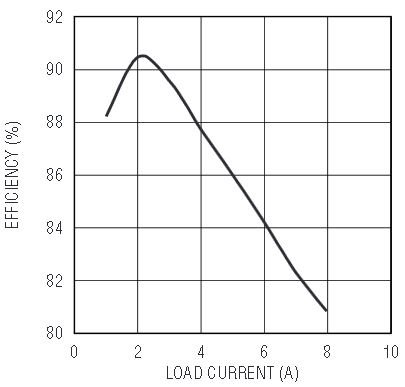Figure 4b. Efficiency vs load current for the circuit shown in Figure 4a, VIN = 3.3V, VTT = 1.25V.

2-Phase 30A VTT Power Supply with a Wide 5V–14V Input Range

In applications with large DDR memory banks, the VTT supply current may exceed 20A. The PolyPhase technique becomes more desirable because it significantly reduces the size of the input and output capacitors. Figure 5 shows a 30A design example using LTC1629, a PolyPhase current mode synchronous buck controller. By tying AMPMD pin of the LTC1629 to INTVCC, we can convert the internal differential amplifier into a real OP AMP that enables the tracking of the output of VDD/2. A 20mV offset is added into both current sense loops through RA1–RA8 to enable the sinking current capability. With only six SO-8 FETs, this circuit is 83% efficient at 5V input, 1.25V/30A output. The same design can be extended to higher input voltages by using input capacitors with higher voltage ratings. As shown in Table 1, the 2-phase technique reduces the total input capacitance by 40% over the conventional single-phase technique. The reduction of the output ripple current also helps achieve a better load transient response.Figure 5. 2-Phase 30A LTC1629 VTT power supply with 5V–14V input.
 Max Input Ripple Current (ARMS) Max Output Ripple Current (AP–P) # Input Capacitors OSCON 16SP270M 1-Phase 13 4.6 5 2-Phase 7.5 4.2 3

Conclusion

The optimum circuit topology for a DDR termination power supply is a synchronous buck converter that can track a reference input voltage while both sourcing and sinking load current. ADI's family of synchronous buck controllers — including the LTC3717, LTC3718, LTC3831 and LTC1629 — provide that functionality along with other features geared towards improving DDR termination power supplies. Table 2 provides a summary of the four DDR termination power supply designs presented in this article, organized by input voltage range and maximum output current. These designs can also be used for QDR (Quadruple Data Rate) applications.

 LTC3718 LTC3717 LTC3831 LTC1629 Input Voltage Range 1.5V–5V 5V–35V or <5V with 5V bias available 3V–8V 5V–35V or <5V with 5V bias available Max output current 20A 20A 20A 40A Reference Schematic Diagram Figure 2 Figure 3 Figure 4 Figure 5